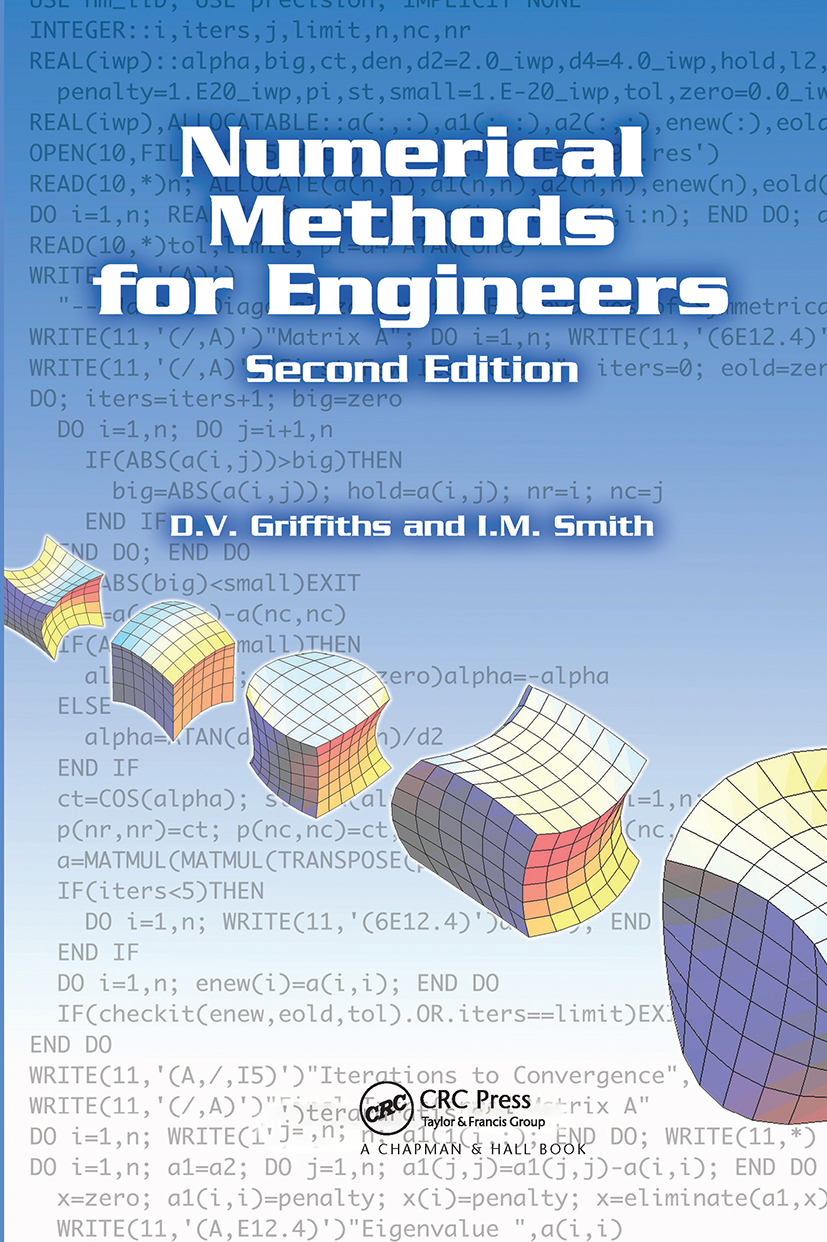# Numerical Methods for Engineers

## 2nd Edition

Chapman and Hall/CRC

492 pages | 83 B/W Illus.

##### Purchasing Options:\$ = USD
Hardback: 9781584884019
pub: 2006-06-22
\$110.00
x
eBook (VitalSource) : 9780429149214
pub: 2006-06-22
from \$28.98

FREE Standard Shipping!

### Description

Although pseudocodes, Mathematica®, and MATLAB® illustrate how algorithms work, designers of engineering systems write the vast majority of large computer programs in the Fortran language. Using Fortran 95 to solve a range of practical engineering problems, Numerical Methods for Engineers, Second Edition provides an introduction to numerical methods, incorporating theory with concrete computing exercises and programmed examples of the techniques presented.

Covering a wide range of numerical applications that have immediate relevancy for engineers, the book describes forty-nine programs in Fortran 95. Many of the programs discussed use a sub-program library called nm_lib that holds twenty-three subroutines and functions. In addition, there is a precision module that controls the precision of calculations.

Well-respected in their field, the authors discuss a variety of numerical topics related to engineering. Some of the chapter features include…

• The numerical solution of sets of linear algebraic equations

• Roots of single nonlinear equations and sets of nonlinear equations

• Numerical quadrature, or numerical evaluation of integrals

• An introduction to the solution of partial differential equations using finite difference and finite element approaches

Describing concise programs that are constructed using sub-programs wherever possible, this book presents many different contexts of numerical analysis, forming an excellent introduction to more comprehensive subroutine libraries such as the numerical algorithm group (NAG).

INTRODUCTION AND PROGRAMMING PRELIMINARIES

Introduction

Running Programs

Hardware

External Fortran Sub-Program Libraries

A Simple Fortran Program

Some Simple Fortran Constructs

Intrinsic Functions

User-Supplied Functions and Subroutines

Errors and Accuracy

Graphical Output

Conclusions

LINEAR ALGEBRAIC EQUATIONS

Introduction

Gaussian Elimination

Equation Solution Using Factorization

Equations with a Symmetrical Coefficient Matrix

Banded Equations

Compact Storage for Variable Bandwidths

Pivoting

Equations with Prescribed Solutions

Iterative Methods

Unsymmetrical Systems

Preconditioning

Comparison of Direct and Iterative Methods

Exercises

NONLINEAR EQUATIONS

Introduction

Iterative Substitution

Multiple Roots and Other Difficulties

Interpolation Methods

Extrapolation Methods

Acceleration of Convergence

Systems of Nonlinear Equations

Exercises

EIGENVALUE EQUATIONS

Introduction

Vector Iteration

Intermediate Eigenvalues by Deflation

The Generalized Eigenvalue Problem [K] {x} = ?[M] {x}

Transformation Methods

Characteristic Polynomial Methods

Exercises

INTERPOLATION AND CURVE FITTING

Introduction

Interpolating Polynomials

Interpolation Using Cubic Spline Functions

Numerical Differentiation

Curve Fitting

Exercises

NUMERICAL INTEGRATION

Introduction

Newton-Cotes Rules

Gauss-Legendre Rules

Special Integration Rules

Multiple Integrals

Exercises

NUMERICAL SOLUTION OF ORDINARY DIFFERENTIAL EQUATIONS

Introduction

Definitions and Types of ODE

Initial Value Problems

Boundary Value Problems

Exercises

INTRODUCTION TO PARTIAL DIFFERENTIAL EQUATIONS

Introduction

Definitions and Types of PDE

First Order Equations

Second Order Equations

Finite Difference Method

Finite Element Method

Exercises

APPENDIX A: Descriptions of Library Subprograms

APPENDIX B: Fortran 95 Listings of Library Subprograms